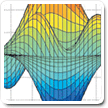# Problem 916. Rubik's Cube : Solve Ten Face Move - Speed Scoring

A set of cubes will be scrambled with 10 Face moves. The Challenge is to Solve all Cubes in 10 Moves or Less. Score will be by cumulative time of cubes 2 thru 11. The first cube may be used to load arrays but is still required to solve the cube. Ten moves relates to worst case 18^10 configs - 3.6e12. In actuality there are only 2.4e11 cubes at 10 turns. Brute force might not work..

Input: Cube vector (1x48](see Figure) with values 0:5, eight of each

Output: Rotation Vector of 10 or less values. Values range from 1:18

Values 1:18 represent the standard cube moves:ufdlbru'f'd'l'b'r'u2f2d2l2b2r2

Example: Move of FL[2 4] requires L'F' solv_vec=[10 8] at a minimum to solve

Cube=[00303003 44410110 11022222 22253533 53344444 11155555] as integer array

solv_vec=[10 8]

Actual solutions will be 8 to 10 values.

The Cube sites claim a capability of solving 10 moves in only 40 hrs. Not sure if they have Matlab, which can achieve 10 moves in <0.15 sec. Matlab with a 16GB/i5 can solve 12 moves in < 1.8 seconds. Unfortunately, Cody appears to have a memory/processing speed issue which makes the 11 and 12 move Challenges impossible.

The next Cube Challenge will be to solve a fully randomized cube in as few moves as possible. With this next challenge I'll post my 3D-Cube Viewer Tool used for algorithm development.

### Solution Stats

25.0% Correct | 75.0% Incorrect
Last Solution submitted on Apr 26, 2023

### Community Treasure Hunt

Find the treasures in MATLAB Central and discover how the community can help you!

Start Hunting!